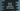# Python rstrip() method explanation with example## Python rstrip() method explanation with example:

Python rstrip method is used to remove or delete trailing characters from a string. It optionally takes an array of characters and removes the trailing characters from the string and returns a new copy of the string.

In this post, we will learn how to use rstrip method with examples.

### Syntax of rstrip:

The syntax of the rstrip method is:

``str.rstrip([chars])``

Here,

• str is the string where we are calling rstrip.
• chars is a string that defines the set of characters to be removed from the end of the string. It is an optional value. If we don’t provide this or if we pass None, it will remove all whitespaces by default.

Note that all combinations of chars are removed. It is not a suffix.

#### Return value of rstrip:

rstrip returns the newly created string.

### Example 1: Without chars:

Let’s try with the below example:

``````first_str = '     Hello      '
second_str = 'Hello\t\n'
third_str = 'Hello        \t   \t   \t'

print(f'first_str = [{first_str}]')
print(f'second_str = [{second_str}]')
print(f'third_str = [{third_str}]')

print(f'\nwith rstrip:\n')
print(f'first_str = [{first_str.rstrip()}]')
print(f'second_str = [{second_str.rstrip()}]')
print(f'third_str = [{third_str.rstrip()}]')``````

Here, we are using rstrip with three different strings: first_str, second_str and third_str.

If you run this program, it will print the below output:

``````first_str = [     Hello      ]
second_str = [Hello
]
third_str = [Hello                              ]

with rstrip:

first_str = [     Hello]
second_str = [Hello]
third_str = [Hello]``````

As you can see here, the rightmost whitespaces are removed from the strings.

### Example 2: With chars:

We can also pass characters as a parameter to rstrip. This is the set of characters that we want to remove from the end of a string.

Let’s take a look at the below example:

``````first_str = '******Hello World******'
second_str = 'Hello8371929371637384911'
third_str = 'youremail@mail.comxyz123'

print(f'first_str = [{first_str}]')
print(f'second_str = [{second_str}]')
print(f'third_str = [{third_str}]')

print(f'\nwith rstrip:\n')
print(f"first_str = [{first_str.rstrip('*')}]")
print(f"second_str = [{second_str.rstrip('123456789')}]")
print(f"third_str = [{third_str.rstrip('123xyz')}]")``````

If you run this, it will print:

``````first_str = [******Hello World******]
second_str = [Hello8371929371637384911]
third_str = [youremail@mail.comxyz123]

with rstrip:

first_str = [******Hello World]
second_str = [Hello]
third_str = [youremail@mail.com]``````

As you can see in these examples, all combinations of the characters are removed from the string, not only the suffix.

### Don’t use rstrip to remove last n characters from a string:

We can’t use rstrip to remove the last n characters from a string. The parameter it takes is not the suffix of the string. It will remove all the combinations of the characters.

Let me show you an example:

``````given_str = 'Hello World !11223211123'

print(given_str.rstrip('123'))``````

In this program, we are trying to remove the last 123 from the string given_str. But, it will delete all the trailing digits because these are either 1 or 2 or 3.

It will print:

``Hello World !``

So, we can’t use rstrip to remove the last n characters from a string.